\$B:#>,\$N0lGU(B

\$BKhF|!":#>,\$N0lGU\$r>e\$2\$F\$\$\$^\$9!#(B
\$B:#>,0{\$`\$b\$N\$K:\$\$C\$?\$i!";29M\$K\$7\$F\$_\$F\$/\$@\$5\$\$!#(B

2023\$BG/(B11\$B7n(B

 1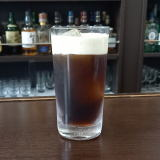(IZ=D%@2Q(B 2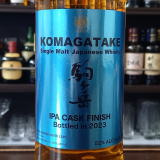\$B6p%v3Y(BIPA(I6=8L(F/<-(B 3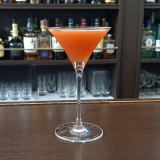(IPX5H1(B 4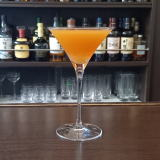(IL^[]8=(B 5 \$B5Y\$_(B 6 \$B5Y\$_(B 7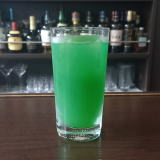(ID[K_6Y%Z2](B 8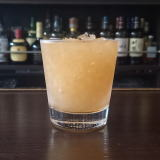(I69^[3(B 9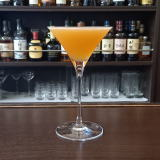(I<,]>^X>^(B 10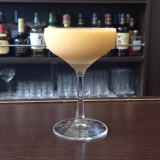(IE2D%7,/L_(B 11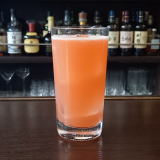(I506^=@(B7 12 \$B5Y\$_(B 13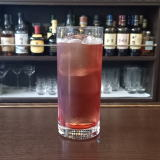(IM^YS/D(B&(I6<=(B 14(I8[/8^%L*=C(J^Y(B 15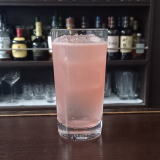(IK_-1%WL^(B 16(IK_6D^0Y(B 17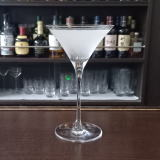(IN\2D%32]8^=(B 18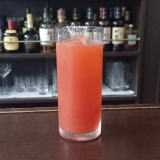(IL^W/C^(%<0;^0(B 19 \$B5Y\$_(B 20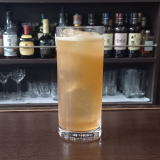(IN^=D]%80W0(B 21(I1L_X:/D%;\0(B 22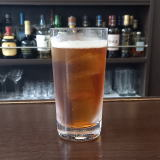(I32=70%:08(B 23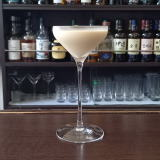(I=^0Q(B 24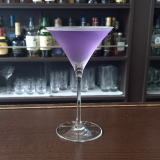(I1<^1]%3*2(B 25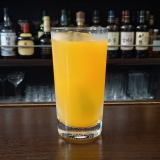(I=8X-0D^W2J^0(B 26 \$B5Y\$_(B 27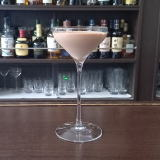(IJ_J_9^0E(B 28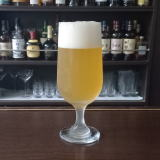(IJ_E<*(B 29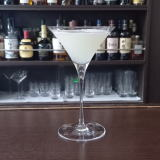(IJ_0L*8D%ZC^((B 30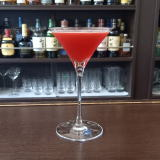(I2]C^(1]%XJ^0(B